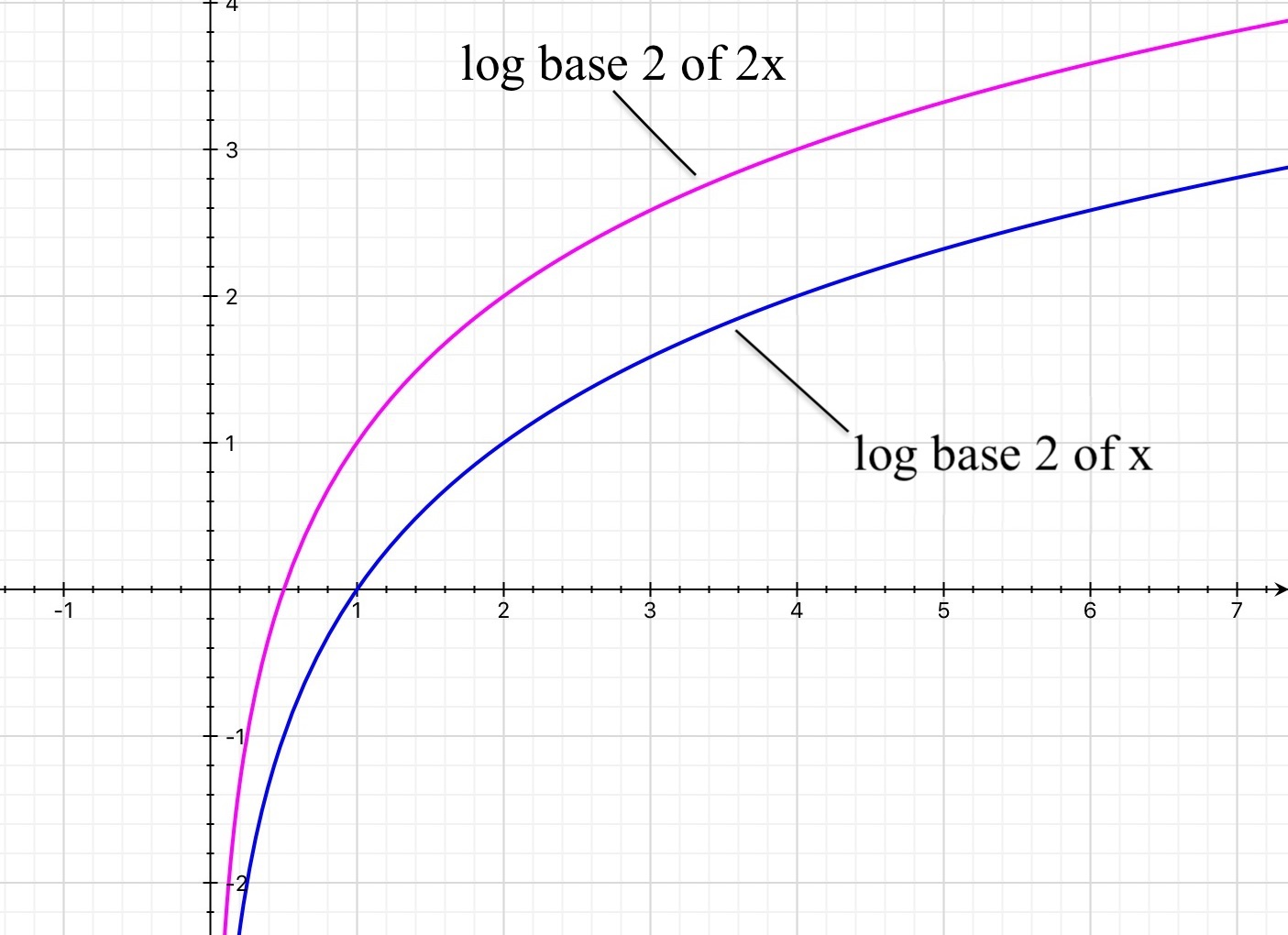# Graphing the Logarithm of a Product

## Questionlog base 2 of x

The graph of the log function  is shown. On the same axes, add the graph of .

## Solution

How will the graph of  compare to the graph of ? Will multiplication by 2 make the graph steeper? Higher?

is the logarithm of a product: . Think of the product rule for logarithms:

This rule applies whether  and  are variables or constants. Then

soTop curve: log base 2 of x
Bottom curve: log base 2 of 2x

Multiplying the argument (that’s x in this case) of a logarithm by a constant increases the value of the logarithm by a fixed amount. That increase is equal to the logarithm of the constant, the number by which you multiply. The two curves have the same shape. The only change is that one is higher than the other.